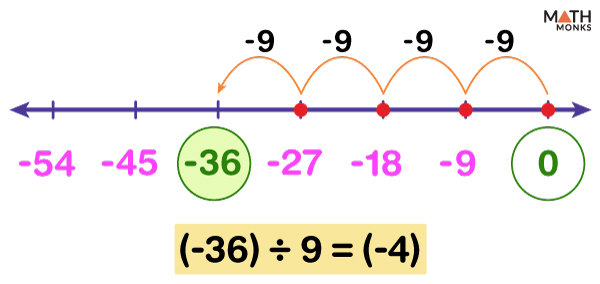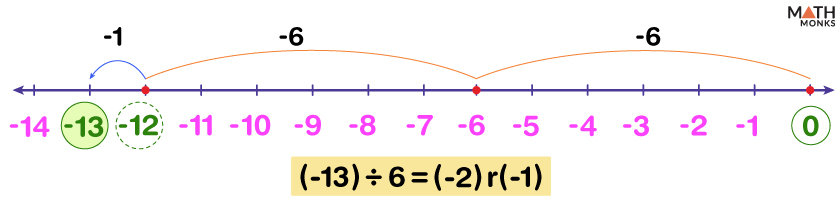# Division on a Number Line

Division on a number line helps us to perform the division operation on a set of numbers and find the result using a number line, given that the numbers are small.

As the division is the same as repeated subtraction, on a number line, the divisor is subtracted from the dividend several times until the result is 0. The number of times the subtraction is repeated to the right or left of 0 gives the quotient as a positive or a negative number.

## With Positive Numbers

When both the dividend and the divisor are positive, we move to the left of the number line, starting from the dividend.

For example, 12 ÷ 4 gives 3, where the dividend is 12, the divisor is 4, the quotient is 3, and the remainder is 0.

Let us find the steps to get the quotient.

Step 1: Both the dividend (12) and the divisor (4) being positive numbers, a positive number line is drawn by plotting the first few multiples of the divisor starting from 0, including the dividend.

Here we draw a number line from 0 to 14, including the first two multiples of 4 and the dividend, 12.

Step 2: For subtraction on a number line, we move to the left of the minuend. However, division starts from the dividend and counts back the number of steps based on the divisor until we get 0.

Starting at 12, we go backward 4 steps each time until we get 0.

Step 3: The number of times the process is repeated to the right of 0 gives us the quotient.

Here the process is repeated 3 times to the right of 0, so the quotient is +3.

Thus, we obtain the quotient 12 ÷ 4 = 3.

Alternative Approach

We can also use an alternative way to perform this division.

After following step 1 above, instead of moving left of the dividend, we move to the right starting from 0 until we get the dividend. The number of steps to go forward each time is decided according to the divisor. Here also, the quotient will be given by the number of times the process is repeated to the right of 0.

For the above example, starting at 0, we move 4 steps three times to the right of 0 to get the dividend, 12. Thus the quotient is +3.

Calculate 20 ÷ 5 using a number line.

Solution:Here, the dividend 20 and the divisor 5 being positive, a positive number line is drawn starting from 0, including the first few multiples of 5.
Next, we move 5 steps to the left each time, starting from 20 until we get 0.
We repeat the above step 4 times to the right of 0 to get the quotient 20 ÷ 5 = 4.

## With Negative Numbers

Here, we can have two possible situations.

Case 1: Negative by a Positive Number

For example, to find (-56) ÷ 8 on a number line,

Step 1: Here, the dividend (-56) being negative, a negative number line is drawn by plotting the first few negative multiples of the divisor 8, including (-56), to the left of 0.

Step 2: Starting from 0, we count back 8 steps each time to get (-56).

Step 3: The same step is repeated seven times to the left of 0 to get the quotient -7.

Thus we get (-56) ÷ 8 = (-7)

Also, as we know, a negative number divided by a positive number gives a negative number; here, the result (-7) is negative.

Locate (-36) ÷ 9 on a number line.

Solution:As the dividend (-36) is negative, a negative number line starting from 0 and with the first few negative multiples of 9 is drawn. Next, we move 9 steps each time to the left of 0 to reach (-36).
Since the process is repeated 4 times to the left of 0, we get the quotient (-36) ÷ 9 = (-4)

Case 2: Negative by a Negative Number

As we know, dividing a negative number by a negative number gives a positive number; here, the steps are the same as dividing a positive number by a positive number.

Let us find (-63) ÷ (-7) on a number line.

Step 1: A number line is drawn starting from 0, including the first few positive multiples of 7.

Step 2: Starting from 63, we move backward by 7 steps each time until we get 0.

Step 3: Since the above process is repeated 9 times to the right of 0, the result is 9.

So (-63) ÷ (-7) = 63 ÷ 7 = 9

Plot the result of (-84) ÷ (-12) on a number line.

Solution:Here the result of the division problem will be positive. So,a positive number line is drawn starting at 0 and with the first few positive multiples of 12, including 84.
Next, we count back 12 steps each time starting from 84 until we get 0. As the process is repeated 7 times to the right of 0, the result is (-84) ÷ (-12) = 84 ÷ 12 = 7.

## With Remainders

Sometimes the dividend is not a multiple of the divisor (i.e., the divisor is not a factor of the dividend). In such cases, the divisor will not completely divide the dividend, and there will be a non-zero remainder.

Let us divide 10 by 4,

Step 1: First, a positive number line with numbers from 0 to at least 10 is drawn.

Step 2: Since the divisor is 4, we start to move 4 steps each time to the right starting from 0 to reach to 10

Step 3: Only 2 jumps of size 4 each from 0 to 8 (one jump from 0 to 4, another from 4 to 8) are taken. Here, the 3rd jump will take us to 12, 2 units more than the dividend.

We are now left with 2 units from 8 to 10.

Thus the quotient is 2 as there are 2 jumps to the right of 0 of equal size 4, and the remainder is 2.

Thus 10 ÷ 4 = 2r2, where r is the remainder.

Find the result (-13) ÷ 6 using a number line.

Solution:As the dividend (-13) is negative, a negative number line starting from 0 and up to at least (-13) is drawn here. We move to the left, starting from 0 by 6 steps each time to reach the dividend (-13). There are 2 such jumps of (-6) and (-1) unit left over.
So the quotient is (-2) because there are 2 jumps of (- 6) to the left of 0, and the remainder is (-1)
Thus the result is (-13) ÷ 6 = (-2)r(-1), r is the remainder.

## With Fractions

While dividing two fractions using a number line, the fraction after the ÷ sign is reversed and ÷becomes ×. Then the multiplication rule is followed.

For example, to calculate  ${\dfrac{2}{5}}$  ÷  ${\dfrac{4}{3}}$ , we write it as ${\dfrac{2}{5}}$  × ${\dfrac{3}{4}}$ and perform the multiplication.

${\dfrac{2}{5}}$  × ${\dfrac{3}{4}}$ = ${\dfrac{2}{5}}$ of ${\dfrac{3}{4}}$

Step 1: As 0 < ${\dfrac{3}{4}}$  < 1, a number line from 0 to 1 is divided into 4 equal parts

Step 2: Each of the three parts from 0 to ${\dfrac{1}{4}}$, ${\dfrac{1}{4}}$ to ${\dfrac{2}{4}}$  and ${\dfrac{2}{4}}$  to  ${\dfrac{3}{4}}$  are shown.

Step 3: As the denominator of the first fraction ${\dfrac{2}{5}}$ is 5, each of the 4 parts is again subdivided into 5 equal parts. Now, there are a total of 20 parts.

Step 4: Since the numerator of the first fraction ${\dfrac{2}{5}}$  is 2, we highlight 2 of each subdivided part from 0 to ${\dfrac{3}{4}}$

This is ${\dfrac{2}{5}}$  of ${\dfrac{3}{4}}$

Step 5: Finally, there are a total of 20 parts. So we can write the first two fractions between 0 and ${\dfrac{1}{4}}$ as ${\dfrac{2}{20}}$ or 2 parts of a total of 20 parts.

Similarly the first two fractions between ${\dfrac{1}{4}}$  and ${\dfrac{2}{4}}$ is also written as ${\dfrac{2}{20}}$ or 2 parts of total 20 parts.

For the last part also, the same is followed.

Adding the three fractions we get the result ${\dfrac{6}{20}}$ , i.e., ${\dfrac{3}{10}}$. So, ${\dfrac{2}{5}}$  ÷  ${\dfrac{4}{3}}$  = ${\dfrac{3}{10}}$.